# Sample Data: Paracou Kimboto Trees

Locations of Kimboto trees at Paracou in French Guiana, annotated with adult / juvenile marks

## Details

Locations of Kimboto trees at Paracou in French Guiana in the observation region Rectangle[{0., 0.}, {400.857, 524.404}] meters, annotated with adult / juvenile marks.

## Examples

### Basic Examples (2)

Retrieve the data:

 In:=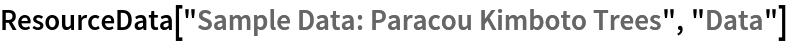Out=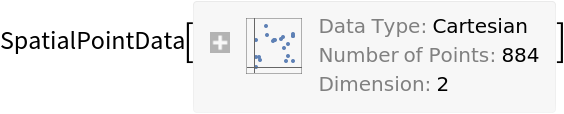Summary of the spatial point data:

 In:=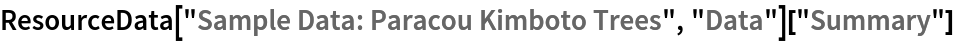Out=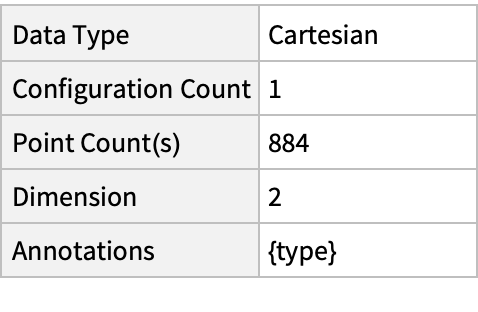### Visualizations (3)

Plot the spatial point data:

 In:=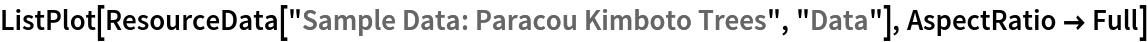Out=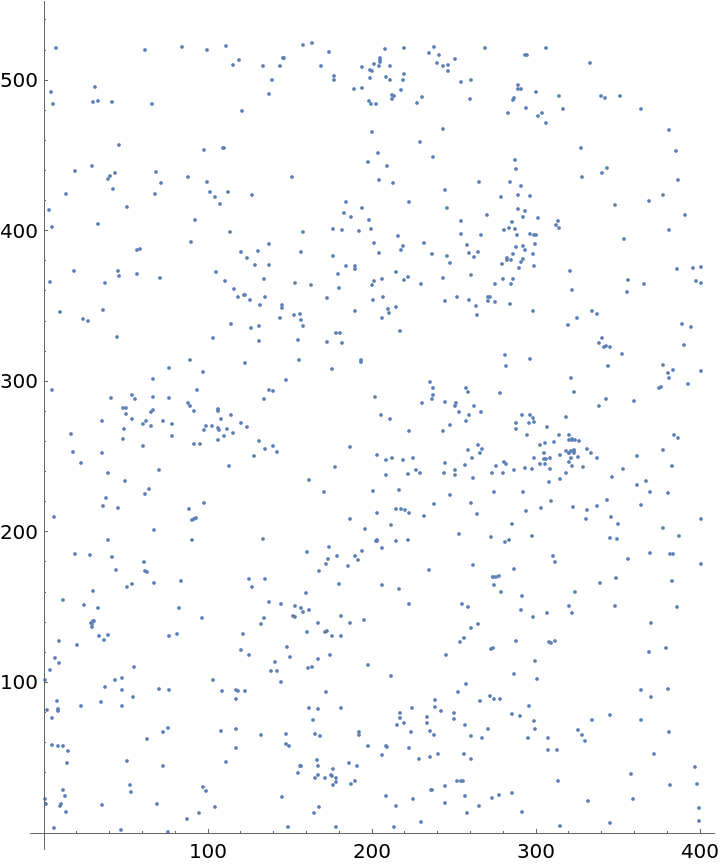Visualize data with annotations:

 In:=Out=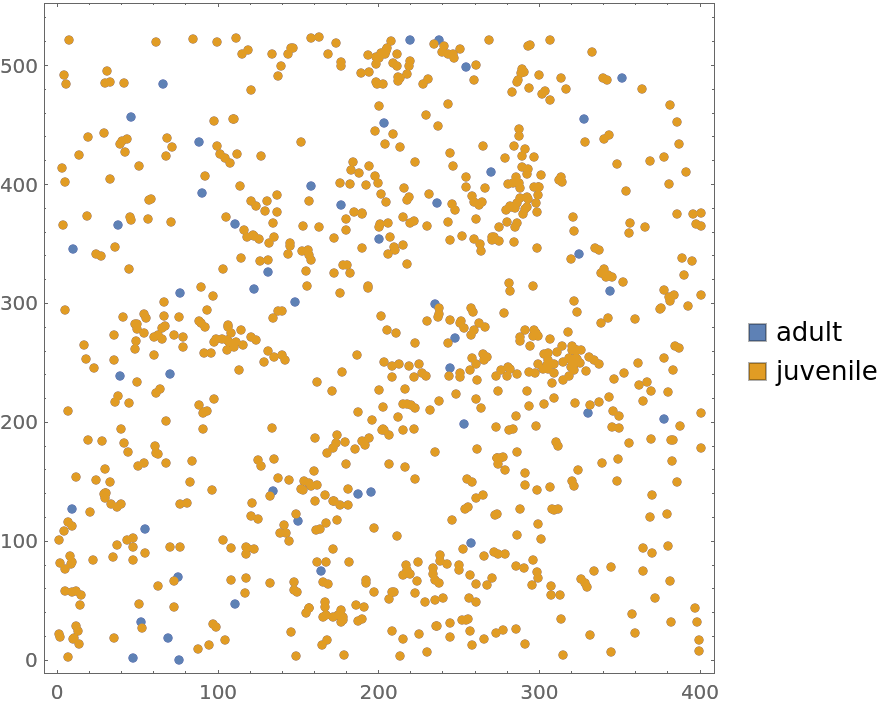Plot the smooth point density:

 In:=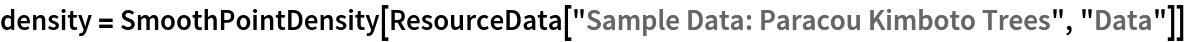Out=In:=Out=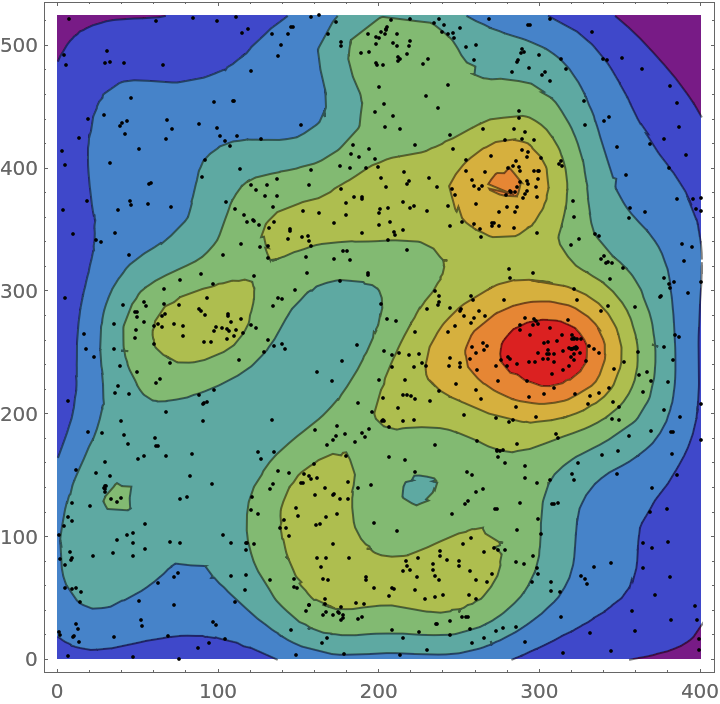### Analysis (3)

Compute probability of finding a point within given radius of an existing point - NearestNeighborG is the CDF of the nearest neighbor distribution:

 In:=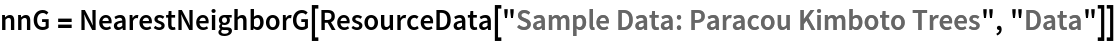Out=In:=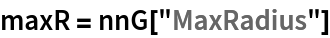Out=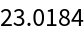In:=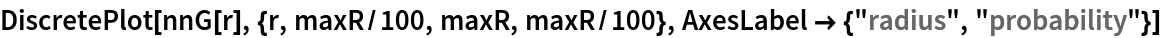Out=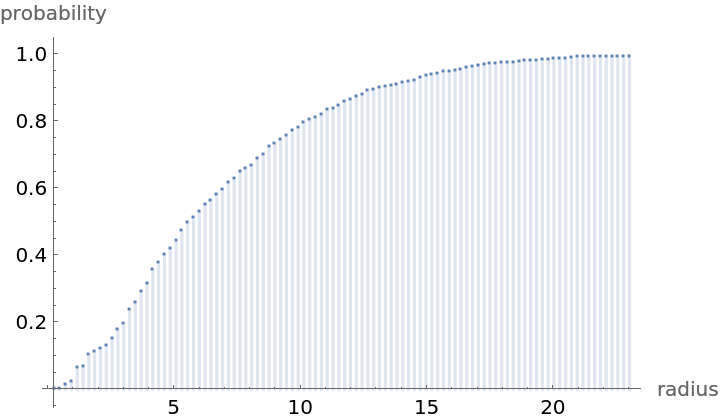Mean distance between a typical point and its nearest neighbor (for positive support distribution can be approximated via a Riemann sum of 1-CDF):

 In:=In:=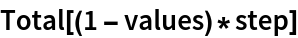Out=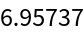Test for complete spacial randomness:

 In:=Out=Gosia Konwerska, "Sample Data: Paracou Kimboto Trees" from the Wolfram Data Repository (2021)